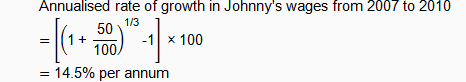Annualised Percentage Growth Concepts and Definitions
PERCENTAGES

## Concepts and Definitions

The annualised percentage growth of a variable over a given period of time refers to the equivalent average rate of growth per annum that will give us the same growth rate as that observed over the entire time span.

Suppose the value of commodity X was 100 in year 2013, rising by 10% to 110 in year 2014 and another 20% to 132 in year 2015.

The annualised rate of growth for commodity X over the 2-year period is 14.9% per annum (p.a.), as this is the equivalent annual rate of growth that will also result in the value of commodity X increasing from 100 in year 2013 to 132 in year 2015.

Annualised and Actual Growth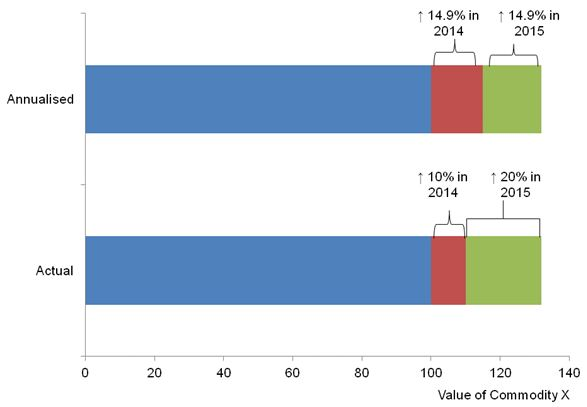How to compute annualised rate of growth?

(1) Given beginning and end levels

Let:
X1 be the level at the beginning of the period
X2 be the level at the end of the period
t be the length of the time period (in years)
r be the annualised rate of growth (in per cent per annum)

Then the annualised rate of growth, r is: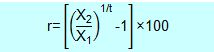Example 1
There were 1,928,300 residents in the labour force in June 2008 and 2,047,300 residents in the labour force in June 2010.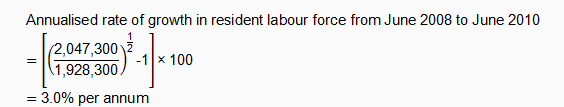(2) Given growth rates for the respective years

Let:
r1 be the growth rate in year 1 (in per cent)
r2 be the growth rate in year 2 (in per cent)
rt be the growth rate in year t (in per cent)
t be the length of the time period (in years)
r be the annualised rate of growth (in per cent per annum)

Then the annualised rate of growth, r is: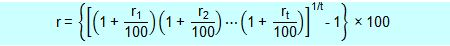Example 2
Real total wages (including employer CPF contributions) fell by 1.0% in 2009, rose by 2.9% in 2010, and rose by 0.9% in 2011.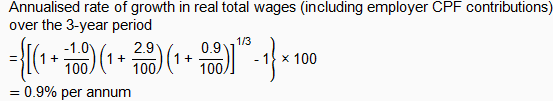(3) Given the cumulative growth rate for the entire period

Let:
R be the cumulative growth rate for the entire period (in per cent)
t be the length of the time period (in years)
r be the annualised rate of growth (in per cent per annum)

Then the annualised rate of growth, r is: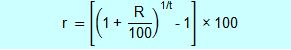Example 3

Johnny’s wages in 2010 was 50% higher than in 2007.